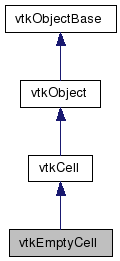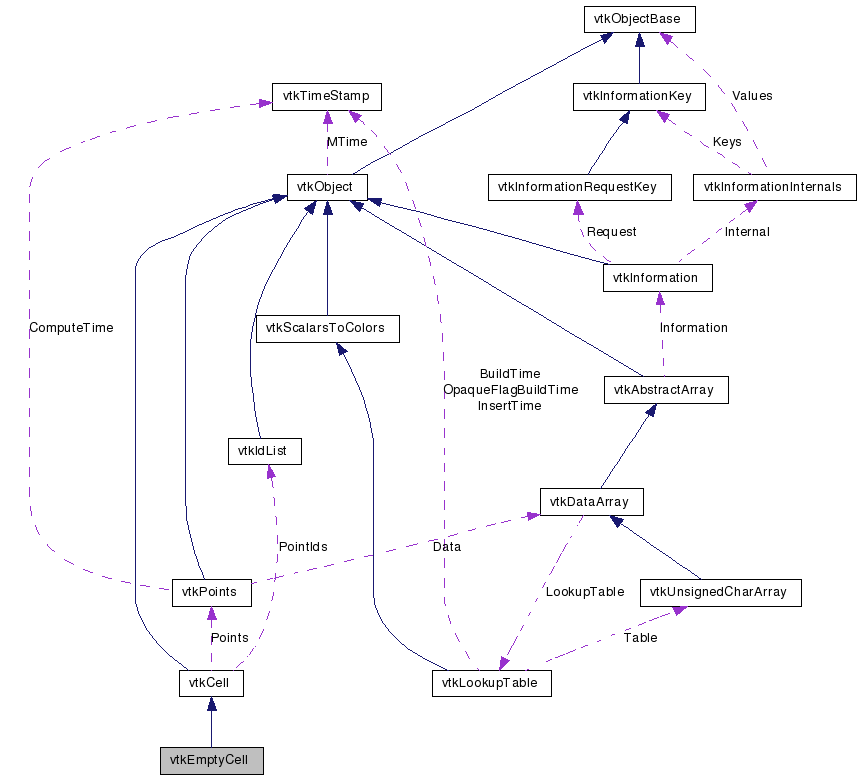# vtkEmptyCell Class Reference

`#include <vtkEmptyCell.h>`

Inheritance diagram for vtkEmptyCell:[legend]
Collaboration diagram for vtkEmptyCell:[legend]

## Detailed Description

an empty cell used as a place-holder during processing

vtkEmptyCell is a concrete implementation of vtkCell. It is used during processing to represented a deleted element.

Tests:
vtkEmptyCell (Tests)

Definition at line 30 of file vtkEmptyCell.h.

## Public Types

typedef vtkCell Superclass

## Public Member Functions

virtual const char * GetClassName ()
virtual int IsA (const char *type)
void PrintSelf (ostream &os, vtkIndent indent)
int EvaluatePosition (double x, double *closestPoint, int &subId, double pcoords, double &dist2, double *weights)
void EvaluateLocation (int &subId, double pcoords, double x, double *weights)
int IntersectWithLine (double p1, double p2, double tol, double &t, double x, double pcoords, int &subId)
int Triangulate (int index, vtkIdList *ptIds, vtkPoints *pts)
void Derivatives (int subId, double pcoords, double *values, int dim, double *derivs)
int GetCellType ()
int GetCellDimension ()
int GetNumberOfEdges ()
int GetNumberOfFaces ()
vtkCellGetEdge (int)
vtkCellGetFace (int)
int CellBoundary (int subId, double pcoords, vtkIdList *pts)
void Contour (double value, vtkDataArray *cellScalars, vtkPointLocator *locator, vtkCellArray *verts1, vtkCellArray *lines, vtkCellArray *verts2, vtkPointData *inPd, vtkPointData *outPd, vtkCellData *inCd, vtkIdType cellId, vtkCellData *outCd)
void Clip (double value, vtkDataArray *cellScalars, vtkPointLocator *locator, vtkCellArray *pts, vtkPointData *inPd, vtkPointData *outPd, vtkCellData *inCd, vtkIdType cellId, vtkCellData *outCd, int insideOut)
virtual void InterpolateFunctions (double pcoords, double *weights)
virtual void InterpolateDerivs (double pcoords, double *derivs)

## Static Public Member Functions

static vtkEmptyCellNew ()
static int IsTypeOf (const char *type)
static vtkEmptyCellSafeDownCast (vtkObject *o)

## Protected Member Functions

vtkEmptyCell ()
~vtkEmptyCell ()

## Member Typedef Documentation

 typedef vtkCell vtkEmptyCell::Superclass

Reimplemented from vtkCell.

Definition at line 34 of file vtkEmptyCell.h.

## Constructor & Destructor Documentation

 vtkEmptyCell::vtkEmptyCell ( ) ` [inline, protected]`

Definition at line 77 of file vtkEmptyCell.h.

 vtkEmptyCell::~vtkEmptyCell ( ) ` [inline, protected]`

Definition at line 78 of file vtkEmptyCell.h.

## Member Function Documentation

 static vtkEmptyCell* vtkEmptyCell::New ( ) ` [static]`

Create an object with Debug turned off, modified time initialized to zero, and reference counting on.

Reimplemented from vtkObject.

 virtual const char* vtkEmptyCell::GetClassName ( ) ` [virtual]`

Reimplemented from vtkCell.

 static int vtkEmptyCell::IsTypeOf ( const char * name ) ` [static]`

Return 1 if this class type is the same type of (or a subclass of) the named class. Returns 0 otherwise. This method works in combination with vtkTypeRevisionMacro found in vtkSetGet.h.

Reimplemented from vtkCell.

 virtual int vtkEmptyCell::IsA ( const char * name ) ` [virtual]`

Return 1 if this class is the same type of (or a subclass of) the named class. Returns 0 otherwise. This method works in combination with vtkTypeRevisionMacro found in vtkSetGet.h.

Reimplemented from vtkCell.

 static vtkEmptyCell* vtkEmptyCell::SafeDownCast ( vtkObject * o ) ` [static]`

Reimplemented from vtkCell.

 void vtkEmptyCell::PrintSelf ( ostream & os, vtkIndent indent ) ` [virtual]`

Methods invoked by print to print information about the object including superclasses. Typically not called by the user (use Print() instead) but used in the hierarchical print process to combine the output of several classes.

Reimplemented from vtkCell.

 int vtkEmptyCell::GetCellType ( ) ` [inline, virtual]`

See the vtkCell API for descriptions of these methods.

Implements vtkCell.

Definition at line 39 of file vtkEmptyCell.h.

 int vtkEmptyCell::GetCellDimension ( ) ` [inline, virtual]`

See the vtkCell API for descriptions of these methods.

Implements vtkCell.

Definition at line 40 of file vtkEmptyCell.h.

 int vtkEmptyCell::GetNumberOfEdges ( ) ` [inline, virtual]`

See the vtkCell API for descriptions of these methods.

Implements vtkCell.

Definition at line 41 of file vtkEmptyCell.h.

 int vtkEmptyCell::GetNumberOfFaces ( ) ` [inline, virtual]`

See the vtkCell API for descriptions of these methods.

Implements vtkCell.

Definition at line 42 of file vtkEmptyCell.h.

 vtkCell* vtkEmptyCell::GetEdge ( int ) ` [inline, virtual]`

See the vtkCell API for descriptions of these methods.

Implements vtkCell.

Definition at line 43 of file vtkEmptyCell.h.

 vtkCell* vtkEmptyCell::GetFace ( int ) ` [inline, virtual]`

See the vtkCell API for descriptions of these methods.

Implements vtkCell.

Definition at line 44 of file vtkEmptyCell.h.

 int vtkEmptyCell::CellBoundary ( int subId, double pcoords, vtkIdList * pts ) ` [virtual]`

See the vtkCell API for descriptions of these methods.

Implements vtkCell.

 void vtkEmptyCell::Contour ( double value, vtkDataArray * cellScalars, vtkPointLocator * locator, vtkCellArray * verts1, vtkCellArray * lines, vtkCellArray * verts2, vtkPointData * inPd, vtkPointData * outPd, vtkCellData * inCd, vtkIdType cellId, vtkCellData * outCd ) ` [virtual]`

See the vtkCell API for descriptions of these methods.

Implements vtkCell.

 void vtkEmptyCell::Clip ( double value, vtkDataArray * cellScalars, vtkPointLocator * locator, vtkCellArray * pts, vtkPointData * inPd, vtkPointData * outPd, vtkCellData * inCd, vtkIdType cellId, vtkCellData * outCd, int insideOut ) ` [virtual]`

See the vtkCell API for descriptions of these methods.

Implements vtkCell.

 int vtkEmptyCell::EvaluatePosition ( double x, double * closestPoint, int & subId, double pcoords, double & dist2, double * weights ) ` [virtual]`

Given a point x return inside(=1), outside(=0) cell, or (-1) computational problem encountered; evaluate parametric coordinates, sub-cell id (!=0 only if cell is composite), distance squared of point x to cell (in particular, the sub-cell indicated), closest point on cell to x (unless closestPoint is null, in which case, the closest point and dist2 are not found), and interpolation weights in cell. (The number of weights is equal to the number of points defining the cell). Note: on rare occasions a -1 is returned from the method. This means that numerical error has occurred and all data returned from this method should be ignored. Also, inside/outside is determine parametrically. That is, a point is inside if it satisfies parametric limits. This can cause problems for cells of topological dimension 2 or less, since a point in 3D can project onto the cell within parametric limits but be "far" from the cell. Thus the value dist2 may be checked to determine true in/out.

Implements vtkCell.

 void vtkEmptyCell::EvaluateLocation ( int & subId, double pcoords, double x, double * weights ) ` [virtual]`

Determine global coordinate (x) from subId and parametric coordinates. Also returns interpolation weights. (The number of weights is equal to the number of points in the cell.)

Implements vtkCell.

 int vtkEmptyCell::IntersectWithLine ( double p1, double p2, double tol, double & t, double x, double pcoords, int & subId ) ` [virtual]`

Intersect with a ray. Return parametric coordinates (both line and cell) and global intersection coordinates, given ray definition and tolerance. The method returns non-zero value if intersection occurs.

Implements vtkCell.

 int vtkEmptyCell::Triangulate ( int index, vtkIdList * ptIds, vtkPoints * pts ) ` [virtual]`

Generate simplices of proper dimension. If cell is 3D, tetrahedron are generated; if 2D triangles; if 1D lines; if 0D points. The form of the output is a sequence of points, each n+1 points (where n is topological cell dimension) defining a simplex. The index is a parameter that controls which triangulation to use (if more than one is possible). If numerical degeneracy encountered, 0 is returned, otherwise 1 is returned. This method does not insert new points: all the points that define the simplices are the points that define the cell.

Implements vtkCell.

 void vtkEmptyCell::Derivatives ( int subId, double pcoords, double * values, int dim, double * derivs ) ` [virtual]`

Compute derivatives given cell subId and parametric coordinates. The values array is a series of data value(s) at the cell points. There is a one-to-one correspondence between cell point and data value(s). Dim is the number of data values per cell point. Derivs are derivatives in the x-y-z coordinate directions for each data value. Thus, if computing derivatives for a scalar function in a hexahedron, dim=1, 8 values are supplied, and 3 deriv values are returned (i.e., derivatives in x-y-z directions). On the other hand, if computing derivatives of velocity (vx,vy,vz) dim=3, 24 values are supplied ((vx,vy,vz)1, (vx,vy,vz)2, ....()8), and 9 deriv values are returned ((d(vx)/dx),(d(vx)/dy),(d(vx)/dz), (d(vy)/dx),(d(vy)/dy), (d(vy)/dz), (d(vz)/dx),(d(vz)/dy),(d(vz)/dz)).

Implements vtkCell.

 virtual void vtkEmptyCell::InterpolateFunctions ( double pcoords, double * weights ) ` [virtual]`

Compute the interpolation functions/derivatives (aka shape functions/derivatives)

 virtual void vtkEmptyCell::InterpolateDerivs ( double pcoords, double * derivs ) ` [virtual]`

Compute the interpolation functions/derivatives (aka shape functions/derivatives)

The documentation for this class was generated from the following file:

Generated on Wed Jun 3 19:01:05 2009 for VTK by1.5.6